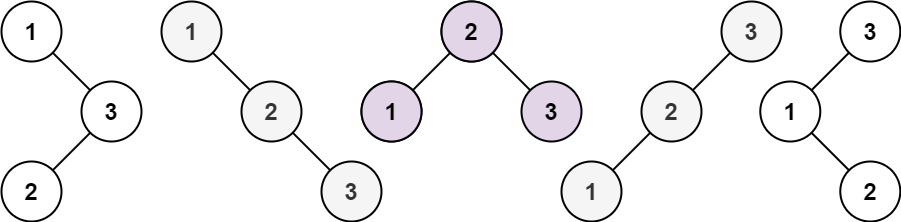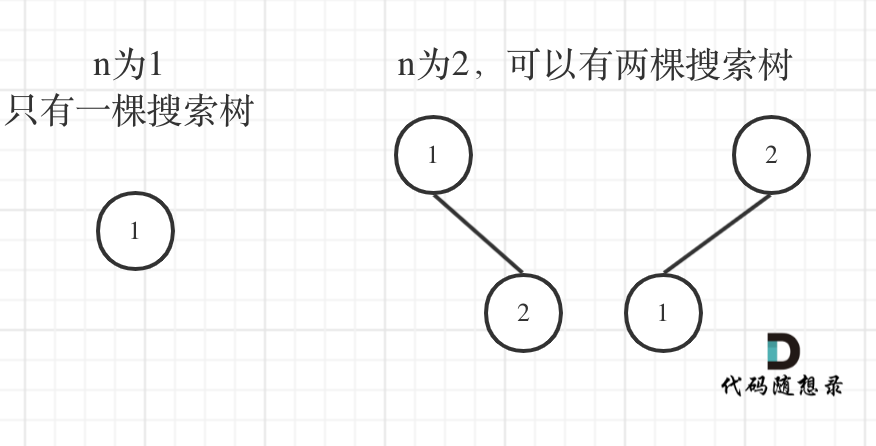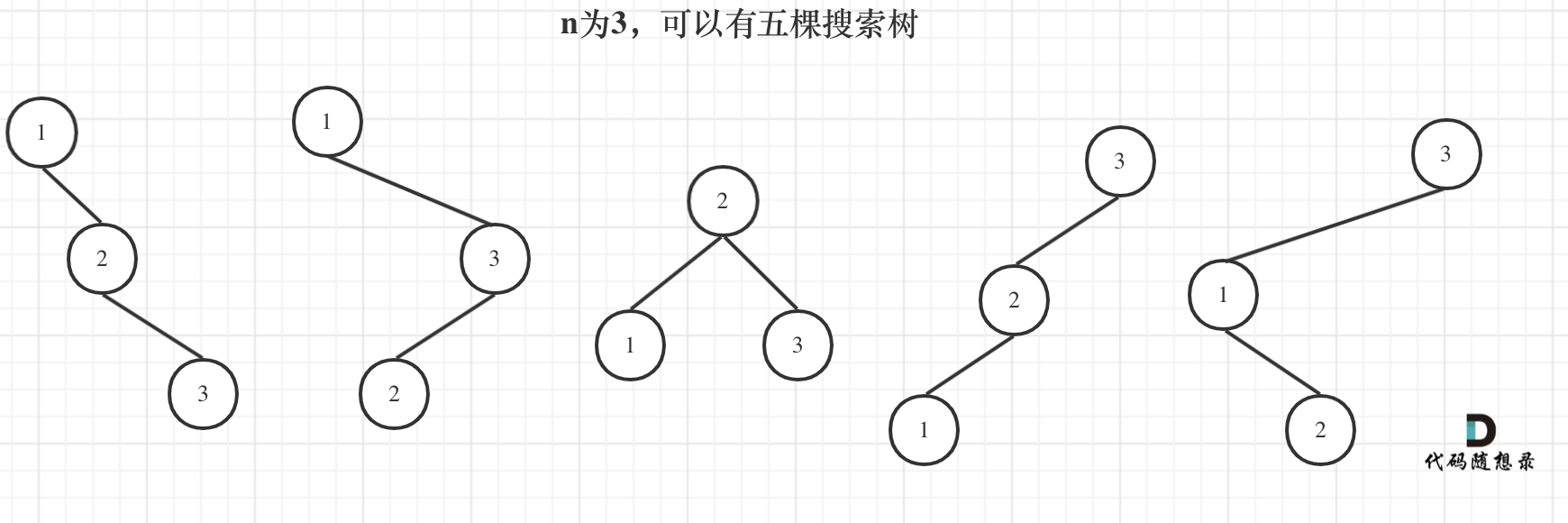# Leetcode 96 - Unique Binary Search Tree

Note:

• May 2022 update
• Forget about DP coz it’s from some math formula, it’s more instinctive to use DFS.
• Remember to use a memo to remember result we’ve got. The key should be left.right.

Given an integer n, return the number of structurally unique BST’s (binary search trees) which has exactly n nodes of unique values from 1 to n.

Example:• DP[i] represents the number of unique BST trees.
• The DP formula is DP[i] += DP[j] + DP[i-j-1].
• Explaination:DP = DP*DP + DP*DP + DP*DP.
• For n=3, when 1 is the root, the number of trees with 1 as the root is DP*DP. When 2 is the root, the number of trees with 2 as the root is DP*DP. When 3 is the root, the number of trees with 3 as the root is DP*DP.